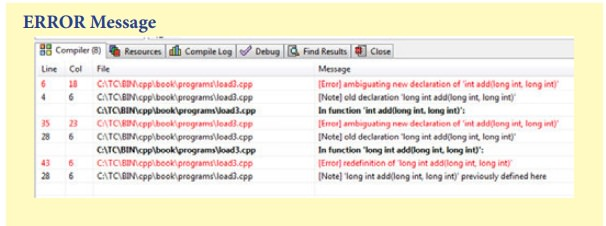## Chapter: 11th Computer Science : Chapter 15 : Polymorphism

The ability of the function to process the message or data in more than one form is called as function overloading.

The ability of the function to process the message or data in more than one form is called as function overloading. In other words function overloading means two or more functions in the same scope share the same name but their parameters are different. In this situation, the functions that share the same name are said to be overloaded and the process is called function overloading . The number and types of a function's parameters are called the function's signature. When you call an overloaded function, the compiler determines the most appropriatedefinition to use, by comparing the argument types you have used to callthe function with the parameter types specified in the definitions. The process of selecting the most appropriate overloaded function or operator is called overload resolution

sometimes it's hard to find many different meaningful names for a single action.

Consider the situation to find the area of circle ,triangle and rectangle the following function prototype is givenfloat area_circle(float radius) // to calculate the area of a circle

float area_triangle(float half,floatbase,float height) // to calculate the area of a triangle

float area_rectangle(float length , float breadth) // to calculate the area of a rectangle

This can be rewritten using a single function header in the following manner

float area ( float half, float base, float height );

float area ( float length , float breadth);

#include <iostream>

using namespace std;

void print(int i)

{cout<< " It is integer " << i <<endl;}

void print(double f)

{ cout<< " It is float " << f <<endl;} void print(string c)

{ cout<< " It is string " << c <<endl;} int main() {

print(10);

print(10.10);

print("Ten");

return 0;

}

### Output:

It is integer 10

It is float 10.1

It is string Ten

1) The overloaded function must differ in the number of its arguments or data types

3) The default arguments of overloaded functions are not considered as part of the parameter list in function overloading.

#include <iostream>

using namespace std;

int main()

{

long a, b, c,d;

float e, f, g;

cout << "Enter three integers\n";

cin >> a >> b>>c;

d=add(a,b,c); //number of arguments different but same data type

cout << "Sum of 3 integers: " << d << endl;

cout << "Enter two integers\n";

cin >> a >> b;

c = add(a, b); //two arguments data type same with above function call and different with below function call

cout << "Sum of 2 integers: " << c << endl;

cout << "Enter two floating point numbers\n";

cin >> e >> f;

g = add(e, f); //two arguments similar to the above function call but data type different

cout << "Sum of floats: " << g << endl;

}

{

long sum;

sum = c + g;

return sum;

}

{

float sum;

sum = c + g;

return sum;

}

long add(long c, long g,long h)

{

long sum;

sum = c + g+h;

return sum;

}

### Output

Enter three integers

3 4 5

Sum of 3 integers: 12

Enter two integers

4 6

Sum of 2 integers: 10

Enter two floating point numbers

2.1 3.1

Sum of floats: 5.2

## Illustration 15.3 Default argument and return type are not considered in overloading

#include <iostream>

using namespace std;

int add(long,long);  // [Error] ambiguating new declaration of 'int add(long int, long int)

int main()

{

long a, b, c;

int d;

cout<< "Enter two integers\n";

cin>> a >> b;

d=add(a,b); //arguments and datatype are same but return is different

cout<< "Sum of 2 integers: " << d <<endl; cout<< "Enter two long integers\n";

cin>> a >> b;

cout<< "Sum of 2 long integers: " << c <<endl;

cout<< "Enter a long integers\n";

cin>> a ;

c = add(a); // [Error] ambiguating new declaration of 'int add(long int, long int)

cout<< "Sum of 2 long integers: " << c <<endl; }

long add(long c, long g) // 'long int add(long int, long int)' previously defined here

{

long sum;

sum = c + g;

return sum;

}

int add(long c, long g)    // [Error] ambiguating new declaration of 'int add(long int,

long int)

{

int sum;

sum = c + g;

return sum;

}

long add(long c, long g=20) // [Error] redefinition of 'long int add(long int, long int)'

{

long sum;

sum = c + g;

return sum;

}

### ERROR MessageStudy Material, Lecturing Notes, Assignment, Reference, Wiki description explanation, brief detail
11th Computer Science : Chapter 15 : Polymorphism : C++ Function overloading |